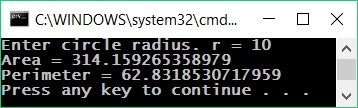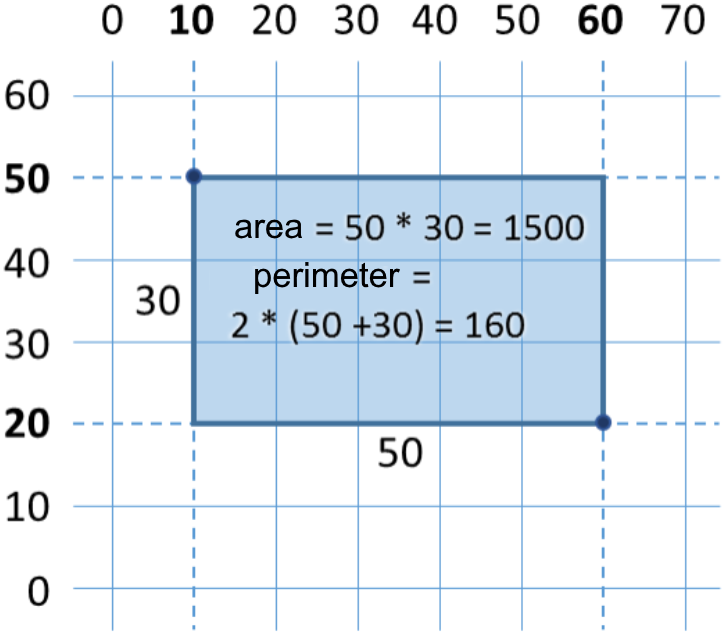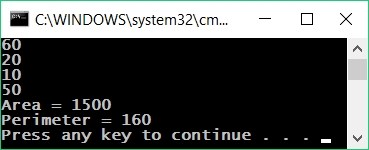# Numerical Expressions

In programming, we can calculate numerical expressions, for example:

var expr = (3 + 5) * (4 – 2);


The standard rule for priorities of arithmetic operations is applied: multiplying and dividing are always done before adding and subtracting. In case of an expression in brackets, it is calculated first, but we already know all of that from school math.

## Video: Numerical Expressions

Watch a video lesson about numerical expressions: https://youtu.be/6MPxlOCsPdw.

## Example: Calculating Trapezoid Area

Let's write a program that inputs the lengths of the two bases of a trapezoid and its height (one floating point number per line) and calculates the area of the trapezoid by the standard math formula:

var b1 = double.Parse(Console.ReadLine());
var b2 = double.Parse(Console.ReadLine());
var h = double.Parse(Console.ReadLine());
var area = (b1 + b2) * h / 2.0;
Console.WriteLine("Trapezoid area = " + area);


If we start the program and enter values for the sides: 3, 4 and 5, we will obtain the following result:

3
4
5
Trapezoid area = 17.5


### Testing in the Judge System

Test your solution here: https://judge.softuni.org/Contests/Practice/Index/504#4.

## Example: Circle Area and Perimeter

Let's write a program that calculates a circle area and perimeter by reading its radius r.

Formulas:

• Area = π * r * r
• Perimeter = 2 * π * r
• π ≈ 3.14159265358979323846…
Console.Write("Enter circle radius. r = ");
var r = double.Parse(Console.ReadLine());
Console.WriteLine("Area = " + Math.PI * r * r);
// Math.PI – built-in constant for π in C#
Console.WriteLine("Perimeter = " + 2 * Math.PI * r);


Let's test the program with radius r = 10:### Testing in the Judge System

Test your solution here:https://judge.softuni.org/Contests/Practice/Index/504#5.

## Example: 2D Rectangle Area

A rectangle is given with the coordinates of two of its opposite angles. Calculate its area and perimeter:In this task, we have to consider that if we subtract the smaller x from the bigger x, we will obtain the length of the rectangle. Identically, if we subtract the smaller y from the bigger y, we will obtain the height of the rectangle. What is left is to multiply both sides. Here is an example of an implementation of the described logic:

var x1 = double.Parse(Console.ReadLine());
var y1 = double.Parse(Console.ReadLine());
var x2 = double.Parse(Console.ReadLine());
var y2 = double.Parse(Console.ReadLine());

// Calculating the sides of the rectangle:
var width = Math.Max(x1, x2) - Math.Min(x1, x2);
var height = Math.Max(y1, y2) - Math.Min(y1, y2);

Console.WriteLine("Area = " + width * height);
Console.WriteLine("Perimeter = " + 2 * (width + height));


We use Math.Max(a, b), to find the higher value from a and b and identically Math.Min(a, b) to find the lower of both values.

When the program is executed with the values from the coordinate system given in the condition, we obtain the following result:### Testing in the Judge System

Test your solution here:https://judge.softuni.org/Contests/Practice/Index/504#6.

## Other Expressions

Expressions in C# can be not only numerical, but also can be text expressions, date expressions or expressions of other type:

var price = 20;
var priceUSD = "$" + price; // text expression var priceGBP = price + " GBP"; Console.WriteLine(priceUSD); //$ 20
Console.WriteLine(priceGBP); // 20 GBP
var date = new DateTime(2017, 6, 14);
var dateAfter5days = date.AddDays(5); // 14-Jun-17 (date expression)
Console.WriteLine(dateAfter5days); // 19-Jun-17 00:00:00